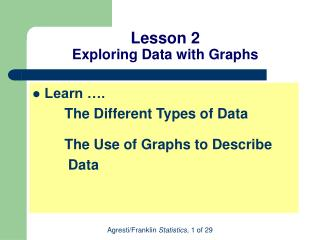# Lesson 2 Exploring Data with Graphs - PowerPoint PPT PresentationDownload PresentationLesson 2 Exploring Data with Graphs

Lesson 2 Exploring Data with Graphs
Download Presentation## Lesson 2 Exploring Data with Graphs

- - - - - - - - - - - - - - - - - - - - - - - - - - - E N D - - - - - - - - - - - - - - - - - - - - - - - - - - -
##### Presentation Transcript

1. Lesson 2Exploring Data with Graphs • Learn …. The Different Types of Data The Use of Graphs to Describe Data

2. Section 2.1 What are the Types of Data?

3. In Every Statistical Study: • Questions are posed • Characteristics are observed

4. Characteristics are Variables A Variable is any characteristic that is recorded for subjects in the study

5. Variation in Data • The terminology variablehighlights the fact that data values vary.

6. Example: Students in a Statistics Class • Variables: • Age • GPA • Major • Smoking Status • …

7. Data values are called observations • Each observation can be: • Quantitative • Categorical

8. Categorical Variable • Each observation belongs to one of a set of categories • Examples: • Gender (Male or Female) • Religious Affiliation (Catholic, Jewish, …) • Place of residence (Apt, Condo, …) • Belief in Life After Death (Yes or No)

9. Quantitative Variable • Observations take numerical values • Examples: • Age • Number of siblings • Annual Income • Number of years of education completed

10. Graphs and Numerical Summaries • Describe the main features of a variable • For Quantitative variables: key features are center and spread • For Categorical variables: key feature is the percentage in each of the categories

11. Quantitative Variables • Discrete Quantitative Variables and • Continuous Quantitative Variables

12. Discrete • A quantitative variable is discrete if its possible values form a set of separate numbers such as 0, 1, 2, 3, …

13. Examples of discrete variables • Number of pets in a household • Number of children in a family • Number of foreign languages spoken

14. Continuous • A quantitative variable is continuous if its possible values form an interval

15. Examples of Continuous Variables • Height • Weight • Age • Amount of time it takes to complete an assignment

16. Frequency Table • A method of organizing data • Lists all possible values for a variable along with the number of observations for each value

17. Example: Shark Attacks

18. Example: Shark Attacks Example: Shark Attacks • What is the variable? • Is it categorical or quantitative? • How is the proportion for Florida calculated? • How is the % for Florida calculated?

19. Example: Shark Attacks • Insights – what the data tells us about shark attacks

20. Identify the following variable as categorical or quantitative: Choice of diet (vegetarian or non-vegetarian): • Categorical • Quantitative

21. Identify the following variable as categorical or quantitative: Number of people you have known who have been elected to political office: • Categorical • Quantitative

22. Identify the following variable as discrete or continuous: The number of people in line at a box office to purchase theater tickets: • Continuous • Discrete

23. Identify the following variable as discrete or continuous: The weight of a dog: • Continuous • Discrete

24. Section 2.2 How Can We Describe Data Using Graphical Summaries?

25. Graphs for Categorical Data • Pie Chart: A circle having a “slice of pie” for each category • Bar Graph: A graph that displays a vertical bar for each category

26. Example: Sources of Electricity Use in the U.S. and Canada

27. Pie Chart

28. Bar Chart

29. Pie Chart vs. Bar Chart • Which graph do you prefer? • Why?